# MA005 Calculus Coaching Session 1.1.1 # 3

Hi this is Swapnila! I am a Student Success Coach and will be explaining some practice problems from the MA005 Calculus course. Today we will review an assigned problem from Unit 1. In the “Homework Assessment” you were asked to solve this problem:
Fig. 9 shows the temperature of a cup of coffee during a ten-minute period. (Each of your answers in (a) – ( c) should have the units “degrees per minute.”)
(a) What was the average rate of cooling from minute 0 to minute 10?
(b) What was the average rate of cooling from minute 7 to minute 8? From minute 8 to minute 9?
(c ) What was the rate of cooling at minute 8? At minute 2?
(d) When was cold milk added to the coffee?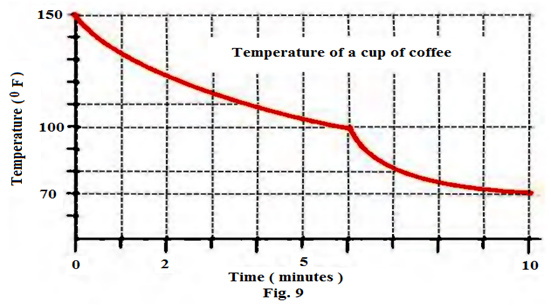Solution:
(a)
Approach this problem as follows:

1. Find temperatures for time minute 0 and time minute 10.
2. Find the average rate of cooling over [a,b] by using the formula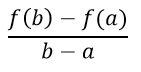Step 1: Find temperatures for time minute 0 and time minute 10:
By using the given graph,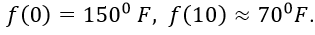Step 2: Find the average rate of cooling:
From step 1, average rate of cooling from minute 0 to minute 10 is calculated by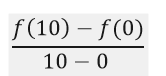Therefore, average rate of cooling becomes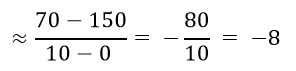Therefore, average rate of cooling becomes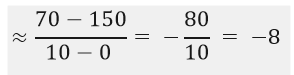Conclusion: The average rate of cooling from minute 0 to minute 10 is approximately 8 degrees per minute.

(b)
Approach this problem as follows:

1. Find temperatures for time minute 7 and time minute 8.
Find temperatures for time minute 8 and time minute 9.
2. Find the average rate of cooling over [a,b] by using the formula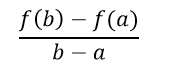Step 1: Find temperatures for time minute 7 and time minute 8:
By using the given graph,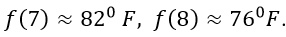By using the given graph,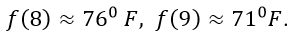Step 2: Find the average rate of cooling:
From step 1, average rate of cooling from minute 7 to minute 8 is calculated by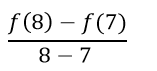Therefore, average rate of cooling becomes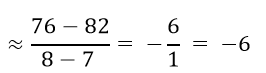From step 1, average rate of cooling from minute 8 to minute 9 is calculated by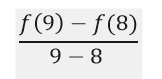Therefore, average rate of cooling becomes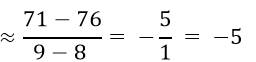Conclusion: The average rate of cooling from minute 7 to minute 8 is approximately 6 degrees per minute. The average rate of cooling from minute 8 to minute 9 is approximately 5 degrees per minute.

( c)
Approach this problem as follows:

1. To get the rate of cooling at minute 8 and minute 2, draw a tangent line at both points. Then choose two
points on each tangent line.
2. Find the slope of the tangent line using the chosen points. Slope of the line passing through (a, f(a)) and
(b, f(b)) is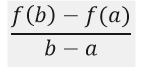Step 1: Draw a tangent line at the given points. Then choose two points on each tangent line:
For minute 8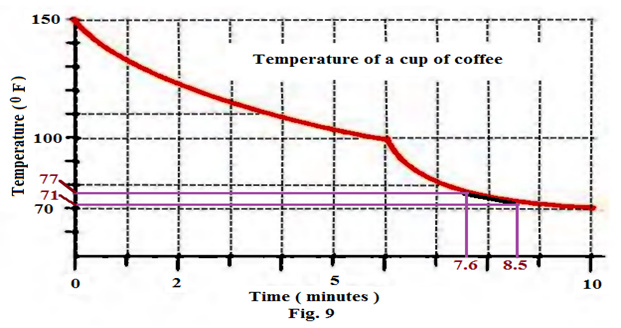For minute 2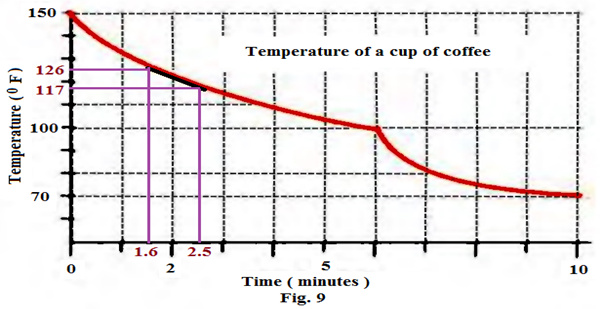Step 2: Find the slope:
For minute 8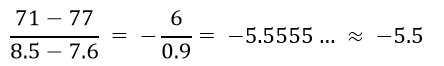For minute 2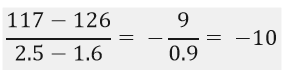Conclusion: The rate of cooling at minute 8 is approximately 5.5 degrees per minute. The rate of cooling at minute 2 is approximately 10 degrees per minute.

(d)
Approach this problem as follows:
Look at the graph, and observe the nature of the curve. Does any sudden change in the temperature or sudden misbehaviour of the curve occur?
The rate of cooling of coffee is smooth up to time 6 minutes, and then there is a sudden change. After 6 minutes, the curve is again smooth. Due to this behavior, cold milk should have been added to the coffee at the time 6 minutes.
Conclusion: Cold milk is added to the coffee at the 6th minute.

In addition to this, I am attaching the pdf document of the practice problem for your ready reference.

Question 3.pdf (371.6 KB)

Please let me know if you have any question on this problem, or on this topic generally. I will be here in the forum for the next hour.

2 Likes

I am new member to this board and just now registered to become a member. I am happy to find this post very useful for me, as it contains lot of information. I always prefer to read the quality content and this thing I found in you post. I think this type of step by step instructions always helpful. It is easy to follow also. I am so happy yo be here. I understand the concept very well. Keep up the good work. For writing tips I use academic writing blog.

How do you get -5.5 for part c at 8 minutes because I came up with -6.6. I did 71-77 which is -6, then I did 8.5 - 7.6 and came up with 0.9. I divide -6 by 0.9 and came up with -6.66666666666, so it about 6.6 .